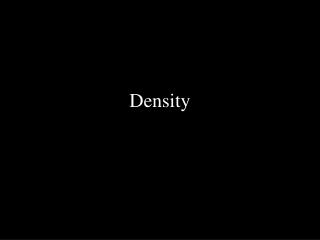DownloadDownload PresentationDensity

# Density

Télécharger la présentation## Density

- - - - - - - - - - - - - - - - - - - - - - - - - - - E N D - - - - - - - - - - - - - - - - - - - - - - - - - - -
##### Presentation Transcript

1. Density

2. What is Density? • Density is the ratio of a material’s mass to its volume. • In other words density is how tightly packed the particles in a material are. • Therefore, D=m/v • D = density (g/mL) • M = mass (grams or g) • V = volume (milliliters or mL)

3. What is Density (contd.) • The density of a material is NOT dependent on how much of the material is present. • That is to say that a handful of copper has the same density as a truckload of copper. • Density is however unique to each material, materials can be identified based on their densities.

4. Experimental Determination of Density • In order to experimentally determine the density of a material the volume and mass must be found. • To find the mass, simply put the material on a balance, lets say we find this material’s mass to be 10g. • Then we must put the material in a graduated cylinder, but 1st we must know how much fluid is in the graduated cylinder • Look for the difference in the fluid

5. Experimental Determination of Density • Suppose the graduated cylinder initially had 10mL in it, then with material it had 15mL in it, what is volume of the material. • 5mL • We then take the mass (10g) and divide it by the volume (5mL) to get the value for density of the material • D = 10g / 5mL = 2g / mL (read as 2 grams per milliliter)

6. Density Problems • You may be asked to determine the volume or mass or density given 2 of the 3 variables. • For help put any equation with 3 variables in this form, A = B x C • Then make the following diagram

7. Use of the Magic Triangle • What you are looking for on the magic triangle, you simply cover up and perform whatever mathematical operation is left.